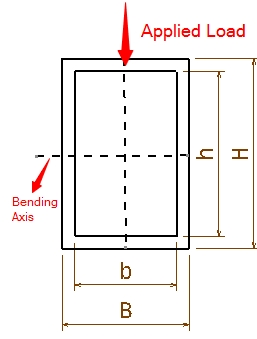This is the Hollow Beam (Rectangular) Moment of Inertia Calculator. Simply enter the length of the two side of the rectangular beam to find the moment of inertia.B = H = b = h = b is the inner length of the side of the rectangle which is always parallel to bending axis.h is the inner length of the side of the rectangle which is always perpendicular to bending axis.B is the outer length of the side of the rectangle which is always parallel to bending axis.H is the outer length of the side of the rectangle which is always perpendicular to bending axis.